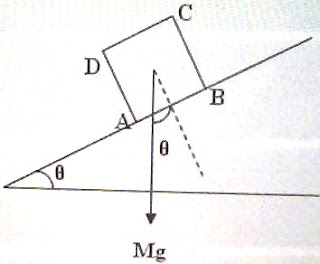## Sunday, June 21, 2009

### IIT-JEE 2009 and Kerala Engineering Entrance 2009 Questions involving Friction

Today we will discuss two questions (MCQ) involving friction. The first one appeared in IIT-JEE 2009 question paper while the second one appeared in KEAM (Engineering) 2009 question paper. Here are the questions with their solution:

(1) A block of base 10 cm × 10 cm and height 15 cm is kept on an inclined plane. The coefficient of friction between them is 3 . The inclination θ of this inclined plane from the horizontal plane is gradually increased form 0º. Then

(a) at θ = 30º, the block will start sliding down the plane

(b) the block will remain at rest on the plane up to certain θ and then it will topple

(c) at θ = 60º, the block will start sliding down the plane and continue to do so at higher angles

(d) at θ = 60º, the block will start sliding down the plane and further increasing θ, it will topple at certain θ.The block will not slide down if the coefficient of friction μ is greater than tan θ. In other words, sliding begins only when θ becomes the angle of friction λ.

Since μ = tan λ we have √3 = tan λ from which λ = 60º.

So the block can start sliding down when θ = 60º.

But the block can topple when the line of action of the weight Mg of the block passes through the edge A of the block (fig.).

This can happen when tan θ = (AB/2) /(BC/2) = AB/BC = 10/15. Therefore, toppling occurs at an angle less than 45º (and greater than 30º).

The correct option therefore is (b).

(2) A mass of 1 kg is just able to slide down the slope of an inclined rough surface when the angle of inclination is 60º. The minimum force necessary to pull the mass up the inclined plane is

(a) 14.14 N

(b)17.32 N

(c) 10 N

(d) 16.66 N

(e) 0.866 N

The angle of friction is given as 60º and hence the coefficient of friction μ is given by

Normally you may assume that the force is applied parallel to the inclined plane. (If the force can be applied without this restriction, the case becomes the most general one and the minimum force necessary to pull the mass up the inclined plane will be less than the value you will get if the force is applied parallel to the plane).

The weight Mg of the mass M acts vertically downwards. The components of this weight along the plane and normal to the plane are Mg sinθ and Mg cosθ respectively. The normal force exerted by the plane on the mass is Mg cos θ and the frictional force is μMg cos θ.

When a force is applied to pull the mass up the plane, the frictional force will oppose the motion and will therefore act down the plane. The total force opposing the tendency of motion of the mass will be Mg sinθ + μMg cos θ.

This is the the minimum force F necessary to pull the mass up the inclined plane.

Substituting proper values, F = (1×10×sin 60º)+ (√3 ×1×10×cos 60º) = 5√3 +5√3 = 17.32 newton [Option (b)].

Note that the mass can be pulled up the plane using a smaller force if the force is applied at an angle α with respect to the plane. The force required will be minimum when α = λ where λ is the angle of friction. The minimum force Fmin is given by

Fmin = Mg sin(θ + λ)

You can find all posts related to friction on this site by clicking on the label ‘friction’ below this post.

Additional questions related to friction can be found here.

1.2.